Courses

# First Law Of Thermodynamic NAT Level - 1

## 10 Questions MCQ Test Topic wise Tests for IIT JAM Physics | First Law Of Thermodynamic NAT Level - 1

Description
This mock test of First Law Of Thermodynamic NAT Level - 1 for IIT JAM helps you for every IIT JAM entrance exam. This contains 10 Multiple Choice Questions for IIT JAM First Law Of Thermodynamic NAT Level - 1 (mcq) to study with solutions a complete question bank. The solved questions answers in this First Law Of Thermodynamic NAT Level - 1 quiz give you a good mix of easy questions and tough questions. IIT JAM students definitely take this First Law Of Thermodynamic NAT Level - 1 exercise for a better result in the exam. You can find other First Law Of Thermodynamic NAT Level - 1 extra questions, long questions & short questions for IIT JAM on EduRev as well by searching above.
*Answer can only contain numeric values
QUESTION: 1

### If R = universal gas constant, the amount of heat needed to raise the temperature of 2 mole of an ideal monoatomic gas from 273K to 373K when no work is done (in units of R)

Solution:

ΔQ = ΔQ = μCVΔT
⇒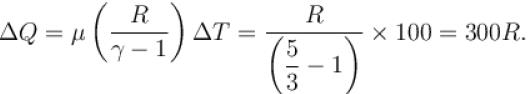*Answer can only contain numeric values
QUESTION: 2

### The weight of a person is 60kg. If he get 105 calories heat through food and the efficiency of his body is 28%, then up to how much height, he can climb (in meters)?

Solution: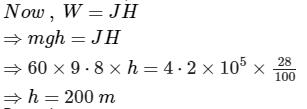*Answer can only contain numeric values
QUESTION: 3

### A thermodynamic process is shown in the figure. The pressure and volumes corresponding to some points in the figure are :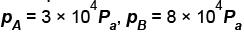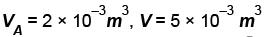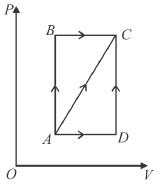In process AB, 600 Joules of heat is added to the system and in process BC, 200J of heat is added to the system. The change in the internal energy of the system in process AC would be? (in Joules)

Solution:

From the graph, WAB = 0
and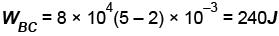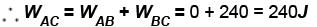Now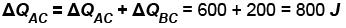From the first law of thermodynamics,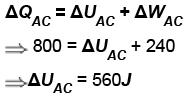*Answer can only contain numeric values
QUESTION: 4

How much energy (in 107 Joules) is absorbed by 10kg molecule of an ideal gas if it expands from its initial pressure of 8 atm to 4 atm at a constant temperature of 27ºC.

Solution: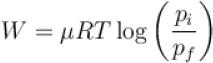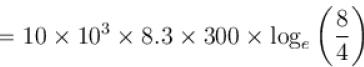W = 1.728×107 J
Amount of energy absorbed = Work done
= 1.728×107J

*Answer can only contain numeric values
QUESTION: 5

A thermodynamic system is taken through the cycle PQRSP process. The net work done by the system is (in Joule)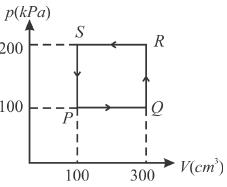Solution:

Work done by the system
= Area of the shaded portion on p-V diagram.
= (300–100)×10-6 × (200–100)×103
= 20J
Since, process is anticlockwise, work done = –20J.

*Answer can only contain numeric values
QUESTION: 6

One mole of O2 gas having a volume equal to 22.4 liters at 0ºC and 1atm pressure is compressed isothermally so that its volume reduces to 11.2 liters. The work done in this process is (in Joules)?

Solution:

Work done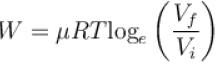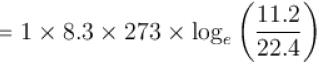= –1572 J

*Answer can only contain numeric values
QUESTION: 7

A gas is compressed at a constant pressure of 50N/m2 from a volume of 10m3 to a volume of 4m3. Energy of 100J is then added to the gas by heating. Then increase in its internal energy is?

Solution:

ΔQ = ΔU + ΔW
⇒ 100 = ΔU + 50(4 - 10)
⇒ ΔU = 100 + 300 = 400J

*Answer can only contain numeric values
QUESTION: 8

A block of mass 100gm slides on a rough horizontal surface. If the speed of the block decreases form 10m/s to 5m/s, then thermal energy developed in the process in Joules is?

Solution:

Thermal energy developed = loss in Kinetic energy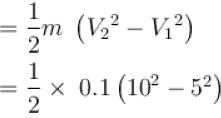= 3.75J

*Answer can only contain numeric values
QUESTION: 9

When an ideal diatomic gas is heated at constant pressure, the fraction of the heat energy supplied which increases. the internal energy of the gas is?

Solution: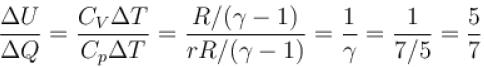*Answer can only contain numeric values
QUESTION: 10

If CV = 4.96 cal/mole-K, then increase in internal energy when temperature of 2moles of this gas is increased from 340K to 342K (in cal)?

Solution:

Increase in internal energy
ΔU = μCVΔT
= 2 × 4.96 × (342 – 340)
= 19.84 cal